## Annotation

If you take any sum, then all other sums can be divided into three groups: identical sums, decomposition sums, and other sums. The classification criteria are the terms and the result of the addition.

## Identical sums

Identical sums are combined into a separate group by the commutativity property. Sums from this group have pairwise identical terms and an equal number of terms. The result of adding these sums is the same. Here is an example of identical sums.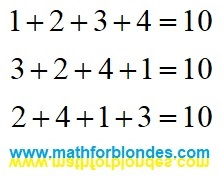Identical sums

The number of sums in this group is determined by the number of terms. For infinite sums, it is equal to infinity.

## Decomposition sums

If the results of adding a different number of terms or terms of different sizes are the same, then such sums form a decomposition group.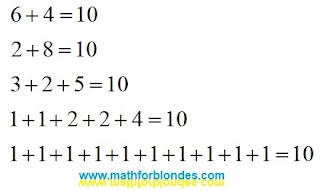Decomposition sums

Any of these sums can be obtained by decomposing the result of addition into terms using linear angular functions. Having thus obtained two terms, any of them can also be decomposed into terms, and so on. An example expansion for the first three sums: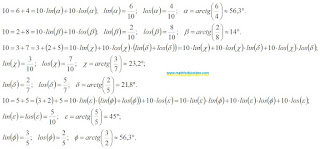Decomposing into terms

An example of decomposing a number into three terms shows that different decomposition options can give the same sum, which underlies the associative properties of addition. The more terms the sum contains, the more different expansion options there can be. The expansion into terms can be continued indefinitely. Different angles and different decomposition algorithms make it possible to obtain different variants of infinite sums. The theory of limits allows you to determine the result of addition based on the analysis of terms. The expansion into terms allows the summation result to be represented as an infinite series of terms.

For example, let's decompose the unit into an infinite sum according to the following principle: the expansion is performed at an angle of 45°, each second term is decomposed into two terms.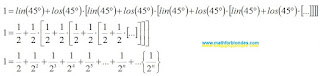Decomposition into an infinite number of terms

The curly brackets indicate the sum of the invisible compensating group of terms, which complements the result of addition to an integer unit. It can be assumed that any sum, even an infinite divergent series, in trigonometric form is equal to one.

## Other sums

If the result of adding any amount differs from the result of adding the amount in question, then this amount has nothing to do with the amount in question and belongs to the “other sums” group.

Let's assume that for the sum a+b=c there is another addition result d that is not equal to c. That is, a+b=d. We represent these two expressions using linear angle functions, and then we translate them into a trigonometric form: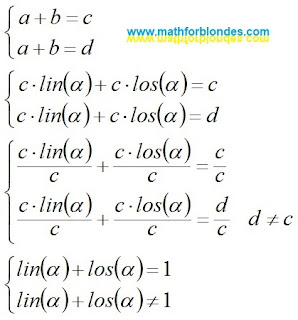Different addition results

The assumption that there are different addition results for the same sum takes us beyond the boundaries of mathematics, where the basic trigonometric relations stop working: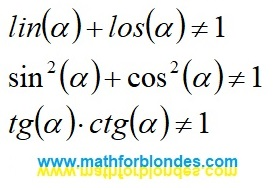Beyond the boundaries of mathematics

Why can't the same sum of terms have two different addition results? You can understand this by considering the reverse process - the transformation of trigonometric functions into specific mathematical sums. I will cover this in more detail in a separate post.

In conclusion of the conversation about the presence of two different results of addition in divergent series, I will give an example from physics. In the earth's crust (convergent series) there are natural caves (the sum of the convergent series). Using special mechanisms (convergence according to Cesaro, etc.) we can get artificial tunnels (the sum of a convergent series). There are no natural caves in the seas and oceans (divergent series, the sum of the series is missing). The use of special mechanisms (convergence according to Cesaro, etc.) allows us to obtain artificial tunnels (the sum of a divergent series) in the water column. Based on this mathematically proven theory, it is possible to design a network of tunnels for roads and railways that encircle the entire earth's surface. Such a theory is quite possible if we do not understand the difference between solids (convergent series) and liquids (divergent series).

## Conclusion

There are no mathematical methods that allow you to get a different addition result for the sum in question.

## Annotation

The use of a shift in arithmetic leads to the addition of terms with different units of measurement.

Consider the results of a shift in arithmetic addition. A shift is possible when performing addition in a column. For example, let's take a three-digit number and add to it the same number without a shift, with a shift by one position and with a shift by two positions. At the same time, we will observe the rule adopted when shifting infinite series - we discard the numbers of the second term that go beyond the boundaries of the first number.Addition with a shift in a column

Shifting by a different number of positions leads to different results in the same way as when shifting infinite series. You can write the same expressions in a line with the subsequent indication of the units of measurement for each of the terms.Addition with a shift in a line

## Conclusion

The shift leads to a change in the unit of measurement of the shifted term. The shift is a violation of the basic law of addition - you cannot add terms with different units of measurement.

## Trigonometry value table for children

In trigonometry value table lists the sines, cosines, and tangents of multiples of 15 degrees from 0 to 90 degrees.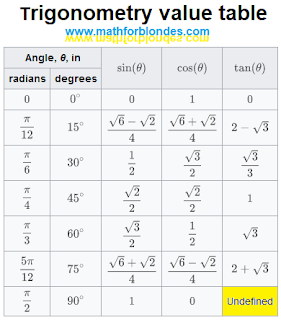Trigonometry value table

Why is this table of trigonometry values for kids (and mathematicians)? Firstly, the values of trigonometric functions are not given in numerical form, but in the form of mathematical expressions using square roots. Secondly, in this table it is indicated that the tangent of 90 degrees is undefined (I specially highlighted this cell in yellow).

The value of the tangent of 90 degrees is determined very simply, but mathematicians, as well as small children, do not understand what this means.

## Division by zero

By definition the tangent of 90 degrees is equal to the sine of 90 degrees divided by the cosine of 90 degrees. Let's take a look at the result.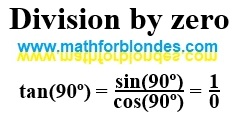Division by zero

The tangent of 90 degrees is equal to one divided by zero. That's what the math says. To understand division by zero, you need to know mathematics much better than we know it. If you firmly believe that zero is a number, you will never understand division by zero. Here you can find some of my publications on mathematics, which are somehow related to division by zero.

Division by zero can be found in various places in mathematics: the sine theorem, the equation of the straight line y=kx and so on. I suggest you explore these formulas on your own. Write about your results in the comments. If necessary, I will show you under what conditions division by zero appears there.

What is division by zero, I will definitely tell you in the future. But before that, there will be many more interesting things.

## Trigonometry value table for engineers

For engineers, a trigonometry table of values should contain numbers so that calculations can be done quickly.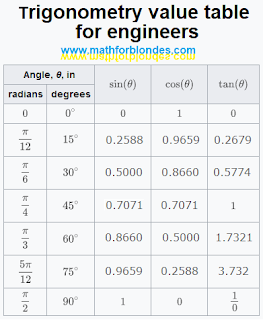Trigonometry value table for engineers

Division by zero is not as important for engineers as it is for physicists. Mathematicians have learned to bypass problem areas in engineering calculations. But physicists in their theories can meet division by zero much more often. If you already want to know what is the result of division by zero in your theoretical research, write in the comments, I will try to help you.

## Annotation

When performing mathematical operations with infinite series, it is necessary to follow the rules of the positional number system.

## Number of terms

The number of terms of an infinite series, presented in the visible part of the series, must be considered as an analogue of writing a number using numbers in the positional number system. The number of terms in the visible part of the series is similar to the rounding of an ordinary decimal fraction with a certain accuracy, that is, to a certain number of decimal places.

When performing mathematical operations with infinite series, for each example it is necessary to use the same number of terms in the visible part of the series. If you do not follow this rule, it can lead to an erroneous result. In mathematics, it is not customary to use any number with different rounding accuracy in one example.

## Shifting series

Shifting an infinite series when performing mathematical operations automatically leads to an incorrect result. This is analogous to adding zeros to the decimal immediately after the decimal point.

Consider an example. If you subtract the same series without a shift from any series, the result will be zero. If the subtraction uses a shift and does not take into account the compensating group of terms, then the result will be different from zero. Suppose we have a series S. Let's write the series -S and add these two series. The result should be zero. By performing addition without shift, we get the correct result.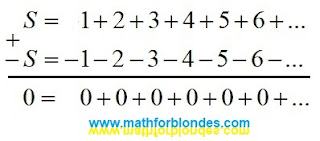Addition of infinite series without shift

Shift by one position leads to wrong result.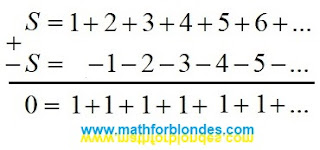Shift by one position

A two-position shift leads to another incorrect result.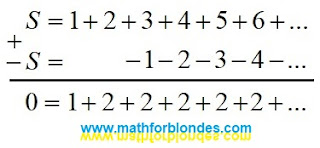A two-position shift

Shifts by an arbitrary number of positions allow you to get an infinite number of incorrect results.

Compliance with the rule about the same number of terms of an infinite series in one example and the use of the compensating group of terms (highlighted in curly brackets) avoids an error. But in the examples given, this method is more laborious than no shift.The use of a compensating group of terms

You can understand the reason for the occurrence of incorrect results if you consider the shift in arithmetic.

Let us analyze some of the most well-known examples of determining the sums of infinite divergent series.

## Sum of Grandi's series

In the given example, the sum of Grandi's series is equal to 1/2, which is not a correct result.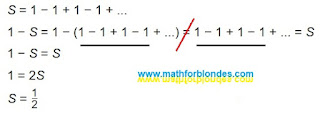Sum of Grandi's series

The second line contains an equals sign between two different sums: one sum consists of five terms that are not equal to zero, the second consists of four. In this example, the optical illusion of the equality of different sums is used, if one term is hidden behind the three-dots of infinity.

## The sum of the alternating series

Below is an alternating series and a solution for determining its sum.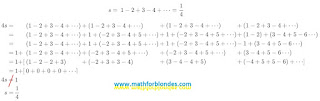The sum of the alternating series

In the proposed solution, the same series is represented by a different number of terms: from four to six. When performing mathematical operations and rearranging terms, the compensating group of terms is ignored. Two other methods for obtaining the sum 4s are not considered: addition of series without shift and multiplication of the original series s by 4. Both these methods give the same result, which indicates the mathematical accuracy of the calculations performed.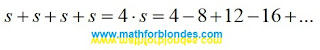The sum 4s

## Sum of natural numbers

Consider the sum of an infinite series of natural numbers. Intuitively, this is a divergent infinite series that cannot have a finite sum value. But, here is an example of calculating the sum of this infinite series.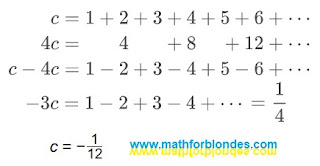Sum of natural numbers

Typical errors of these calculations are shown in the example above. Mathematicians did not check the solution: the original series c, multiplied by minus three, is equal to: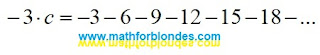Solution verification

## Conclusion

All the above ways of finding the sum of an infinite series are nothing more than fitting the solution to a given result. Nobody prevents mathematicians from establishing their own rules for virtual games in numbers. But the application of such results of "calculations" in the laws of physics leads to their misunderstanding. For example, a numerical coefficient due to some physical parameter or simply a correction factor is interpreted as the sum of some infinite series. An example of an erroneous interpretation of numerical coefficients would be the following statement: the area of ​​a right triangle is equal to the sum of the Grandi series multiplied by the product of its legs.

## 8.12.2022

### Permutation of terms in infinite sums

From the school bench, everyone knows the rule that appeared in antiquity: the amount does not change from the rearrangement of the terms. By default, it is assumed that the number of terms and their value remain unchanged. If we change the number of terms or change the value of at least one of them, there can no longer be any talk of rearranging the terms. In this case, we have moved from one sum to another and these two sums are not connected in any way.

In the article “What is wrong with the rearrangement of terms?” (in Russian) an example of an infinite sum is given, allegedly proving that the final sum depends on the order of addition.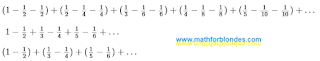Infinite sum

After opening the brackets and reducing the same terms, the result of the addition is different. That's what mathematicians say. Let's consider this example with more careful observance of the rules for writing mathematical expressions.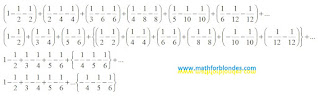Permutation of terms in infinite sums

The first line contains the original infinite sum, consisting of six brackets - three terms in each bracket. The total number of terms is 18, the total sum of the expression is zero. The entire mathematical expression can be divided into two groups: the visible part of the expression, represented by 18 terms, and the invisible part of the expression, consisting of an infinite number of brackets, three terms in each bracket. These two parts are separated by the three-dots of infinity. Both parts are zero.

The second line shows the same 18 terms after the permutation. The first three pairs of terms will be further presented in the visible part of the expression. Curly brackets highlight the compensating group of terms.

In the third line, the six terms of the visible part remain unchanged. The compensating group, after simplifying the expression, is represented by three terms. The final result after rearranging the terms has not changed and is still equal to zero.

The fourth line has nothing to do with mathematics. This is the usual trick of an illusionist who hid the compensating group of terms in the arm of infinity (the invisible part of the expression). The purpose of this trick is to convince gullible viewers of the “truthfulness” of the false statement about the change in the total amount after rearranging the terms. Yes, this is what card cheaters do - they hide the card in their sleeve or take it out of there. In the circus, such a trick is called an illusionist's trick. In legal terms, this is called fraud.

We can also give a cruder example of a permutation of the terms in this expression. If terms with only one sign are shown in the visible part of the expression, the audience will inevitably have a question where the terms with opposite signs have gone, and this will clearly make it difficult to demonstrate the trick.

The permutation of terms in infinite sums clearly demonstrates the principle of communicating vessels. The first vessel is the visible part of the expression. The second vessel is the invisible part of the expression, which includes the compensating group of terms. The three-dots of infinity is the connecting pipe between the vessels. The final sum of the expression is the total volume of liquid in the two vessels. Since in our mathematical example the total sum is equal to zero, then in relation to communicating vessels, we consider the initial total liquid level in the vessels as zero of the relative coordinate system. A change in the number or size of terms in the visible part of the expression will lead to a change in the number or size of terms in the compensating group.

If the last expression is considered without an invisible compensating group of terms, then it will not be the result of a rearrangement of the terms, but a completely different infinite sum, containing only a part of the terms from the original expression and not connected with it in any way. The proof of this fact is the different total sum of the two expressions.

## 8.11.2022

### Multiplication by zero in physics

To finish talking about multiplication by zero, consider an example from physics. There, all laws are written using multiplication. But, unlike mathematicians, physicists understand at least a little what they are talking about.

And so, for simplicity, consider Ohm's law. The simplest option: multiplying current by resistance gives voltage. I specifically use the language and style of mathematicians to describe a physical law. After all, we are trying to understand mathematics. Let's write this in physical notation.

I * R = U

Next, we write a mathematical example of multiplication.

a * b = c

Now find four differences. We have only the appearance of the three letters is different, everything else is the same. If one of the mathematicians begins to assert that the first expression has nothing to do with the second, then I have a question: why is such mathematics needed? Only for cramming? Multiplication perfectly describes physical laws, if by multiplication we mean interaction.

As a result of the interaction of current with resistance, a voltage is obtained. Electricity is given to us by a power plant. Resistance is provided by wires with a light bulb inside the room. The interaction of these physical quantities gives a voltage, which is expressed in the radiance of a light bulb. If there is current and wires, then there is voltage - the bulb is on.Multiplication by zero in physics

Consider the case where multiplication does not occur. The neighbor has electricity, we have wires, but we have no voltage. The light does not burn because the neighbor's current does not interact with our wires and the light bulb. This sad fact is similar to zero in formulas.

I and R = 0

a and b = 0

Now we will consider two cases of multiplication by zero. In the first case, everything is connected correctly, but the power plant does not work. As a result, the lamp does not light up. There is no interaction, no multiplication occurs.

0 * R = 0

0 * b = 0

In the second case, the power plant is working, but we do not have a light bulb. There is no interaction between resistance and current. Again, no multiplication occurs.

I * 0 = 0

a * 0 = 0

As an example from physics shows, multiplication by zero does not occur. The sacred definition of mathematicians "any number multiplied by zero equals zero" is no more than a shaman's lament while dancing with a tambourine.

### Multiplication by zero in geometry

Consider multiplication by zero in geometry. Multiplying the length by the width gives the area. Whatever mathematicians tell you, all the formulas for the areas of any geometric shapes come down to this simple formula. It’s just that for different figures there are different correction factors, but we’ll talk about this some other time.

We have a rectangle and a formula to calculate its area. Traditionally, it is portrayed as follows.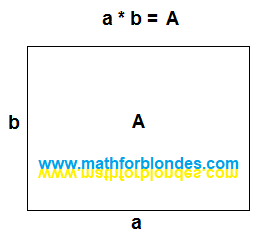Rectangle area

Everything seems to be correct. But... In algebra, we first write down the two factors, then we write the equal sign, and only after that we write down the result of the multiplication. This is a whole series, not just one picture. Let's represent geometrically everything that we write down algebraically. The sides of the rectangle a and b are line segments. The mathematical operation of multiplication has no geometric representation. I will replace the equal sign with a vertical line that divides the picture into two parts - before multiplication and the result of multiplication. The area of rectangle A is, in fact, the rectangle itself. Here's what happened.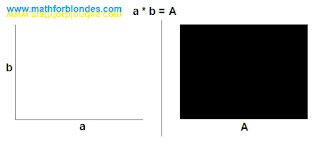Multiplication in geometry

Two perpendicular segments before multiplication turn into the area of a rectangle after multiplication. Now let's draw multiplication by zero.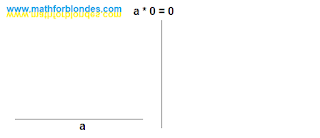Multiplication by zero

As a result of multiplication by zero, we do not get the area. We have the length, but the width is missing. Naturally, the area is simply nowhere to come from. We look at the result of multiplying zero.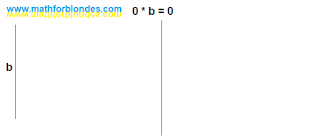Multiplying zero

Now we have a width but no length. Again, the area can not be obtained. Next, we depict the multiplication of zeros - zero multiplied by zero equals zero.Multiplication of zeros

No length, no width, no area. We take nothing, multiply by nothing and get nothing as a result. And now the most interesting.

Let's try to depict geometrically that intimate moment when we do not perform multiplications. Even algebra is bashfully silent about this. How to algebraically write down the fact that we have two segments that can be interpreted as length and width, but we do not perform the mathematical operation of multiplication between them? Not a single fool would come up with such a thing, and fools are not taught this. Sentient beings differ from trained animals in that they can do more than just what their trainers have taught them. We were taught that we perform multiplication only on the command "Milo, multiply!" or when we see the multiplication sign, we simply ignore all other cases. That's why the symbol "do not multiply" is absent in mathematics. I use "and" instead.We don't do multiplication

If we do not perform multiplication, there is no area. Now we can compare the results. If we perform multiplication, the result is an area. If we do not perform multiplication, there is no area.

Conclusion: when operating with zero, the mathematical operation of multiplication is not performed.

It is impossible to perform multiplication with zero - it will not work. It's like sawing air. You pick up a saw, move it back and forth and tell everyone on the phone that you are sawing. Only at the same time you do not specify that you are sawing something that, in principle, it is impossible to saw. By the way, we will consider the physics of multiplication by zero separately.